# Test: Resonance - 1

## 10 Questions MCQ Test Network Theory (Electric Circuits) | Test: Resonance - 1

Description
Attempt Test: Resonance - 1 | 10 questions in 30 minutes | Mock test for Electrical Engineering (EE) preparation | Free important questions MCQ to study Network Theory (Electric Circuits) for Electrical Engineering (EE) Exam | Download free PDF with solutions
QUESTION: 1

### A parallel resonant circuit has a resistance of 2kΩ and half power frequencies of 86 kHz and 90 kHz. Q. The value of capacitor is

Solution: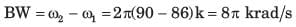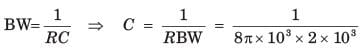= 19.89 nF

QUESTION: 2

### A parallel resonant circuit has a resistance of 2kΩ and half power frequencies of 86 kHz and 90 kHz. Q. The value of inductor is

Solution: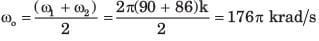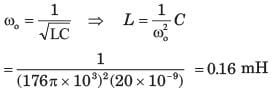QUESTION: 3

### A parallel resonant circuit has a resistance of 2kΩ and half power frequencies of 86 kHz and 90 kHz. Q. The quality factor is

Solution: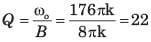QUESTION: 4

A parallel resonant circuit has a midband admittance of 25 x 10-3 S, quality factor of 80 and a resonant frequency of 200 krad s.
Q. The value of R is

Solution:

At mid-band frequency Z = R , Y  = 1/R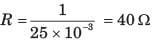QUESTION: 5

A parallel resonant circuit has a midband admittance of 25 x 10-3 S, quality factor of 80 and a resonant frequency of 200 krad s.
Q. The value of C is

Solution:

Q = ω0RC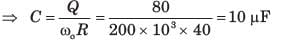QUESTION: 6

A parallel RLC circuit has R = 1kΩ and C = 1 μF. The quality factor at resonance is 200. The value of inductor is

Solution: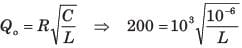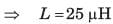QUESTION: 7

A parallel circuit has R = 1kΩ , C = 50 μF and L = 10 mH. The quality factor at resonance is

Solution: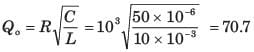QUESTION: 8

A series resonant circuit has an inductor L = 10 mH. The resonant frequency ω0 = 106 rad s and bandwidth is BW = 10rad/s. The value of R and C will be

Solution: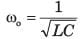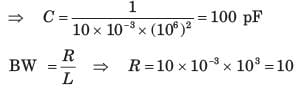QUESTION: 9

A series resonant circuit has L = 1 mH and C = 10 μF. The required R for the BW 15.9 Hz is

Solution: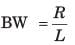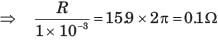QUESTION: 10

For the RLC parallel resonant circuit when R = 8kΩ, L = 40 mH and C = 0.25 μF, the quality factor Q is

Solution: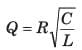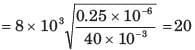Use Code STAYHOME200 and get INR 200 additional OFF Use Coupon Code